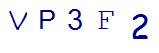# 3 Quick and Easy Ways to Solve 3rd Grade Multiplication Word ProblemsLast Updated on August 31, 2021 by Thinkster

In third grade, math word problems get progressively more challenging. Not only are children asked to complete multi-step problems, but most of those problems now involve multiplication. If your child is struggling with 3rd grade multiplication word problems, here are three strategies that will help them.

## 1. Draw a Picture

Because some of the clue words for multiplication and addition overlap, such as “in all” and “total,” knowing which operation to perform can be difficult for many third graders. Up until third grade, these clue words meant addition. Yet, since multiplication is basically just repeated addition, the clue words overlap.

You can ensure that this is less of an issue by teaching the child to draw a picture of what the problem is saying. So, if the problem says:

Mrs. Smith has 10 boys in her class. Each boy has two pencils. How many pencils do they have in all?

The student can draw 10 stick figures, give them two pencils each. This shows that multiplying 2 x 10 is the way to get the answer. Often the visual representation will show the child that it is, in fact, time to multiply.

## 2. Estimate and Check

Is your child unsure of what to do? Have them estimate by rounding up the numbers, multiplying and then checking if the answer is reasonable. This will allow the child to see if multiplication is the right operation indeed, then go back and do the calculation to get the actual answer. It may seem like it adds time and steps, and in a way it does, but this process eliminates wasted time spent multiplying when the problem calls for addition or subtraction.

## 3. Create a Grid

Grids on graph paper can make multiplication easier to tackle. For multiplication grids, your child should mark off the number of one factor going horizontally on the grid, then the second factor going vertically. Color in the places where the two rows overlap and count the number of squares colored. This is the total product.

If you find that 3rd grade multiplication word problems are still a struggle for your child, even with these quick and handy strategies, then consider investing in some tutoring. To get the most out of your time and investment, consider tutoring that is done on the computer or tablet.

Thinkster Math is an iPad based tutoring program that covers all the topics in? Math curriculum, including 3rd grade multiplication word problems, to give kids a well-rounded instruction at the point of learning. With this program, your child can learn tips and strategies to tackle those challenging word problems and get the right answer consistently.

SummaryArticle Name
3 Quick and Easy Ways to Solve 3rd Grade Multiplication Word Problems
Description
Author
Publisher Name
Thinkster Math
Publisher Logo

## Subscribe to Thinkster Blog## Recommended Articles### Unlocking the Code: Teaching Mathematical Symbols and Their Meaning

Knowledge of mathematical symbols is a foundational skill that empowers students to engage with and excel i...